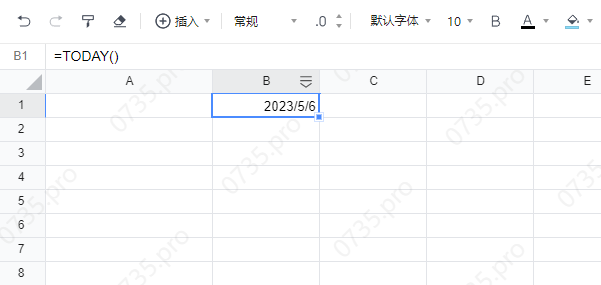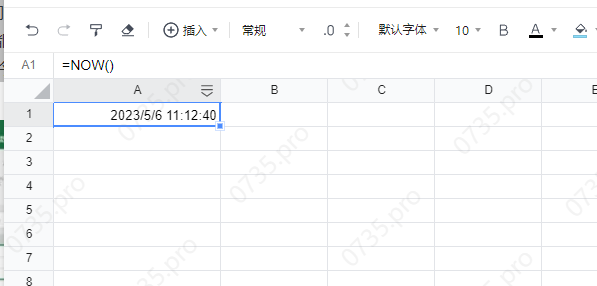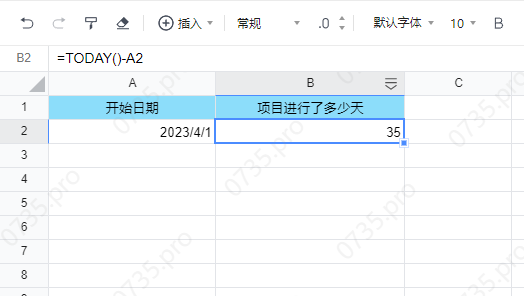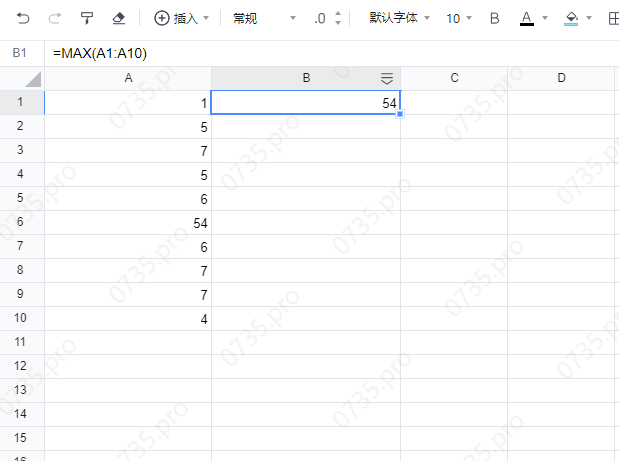excel常用函数公式• 累计撰写 63 篇文章
• 累计收到 0 条评论

# excel常用函数公式2023-05-01 / 0 评论 / 95 阅读 / 正在检测是否收录...

#### SUM函数

SUM函数的作用是求和。函数公式为=sum()例如：

``=sum(A1:A10) ``

``=sum(A1:A10,C1:C10)``

• （+） 代表加
• （-） 代表减
• （/） 代表除
• （*） 代表乘
• （:） 多选单元格

#### AVERAGE函数

Average 的作用是计算平均数。函数公式为=AVERAGE( )例如：

``=AVERAGE(A1:A12)``

#### count函数

``= COUNT(A1:A12)``

#### IF函数：

IF函数的作用是判断一个条件，然后根据判断的结果返回指定值。
IF函数公式为：=IF(逻辑判断,为TRUE时的结果,为FALSE时的结果)

``=IF(A1>A5,1,2)``#### NOW函数和TODAY函数：

NOW函数返回日期和时间，TODAY函数则只返回日期。

NOW函数和TODAY函数都没有参数，只用一对括号即可：`` =TODAY()````=NOW() ``

=TODAY() - 开始日期得出的数字就是项目进行的天，例如：

``=TODAY() - a2``#### VLOOKUP函数

VLOOKUP函数用来在表格中查找数据，函数的用法：

``````=VLOOKUP(查找值,区域,要返回第几列的内容,1近似匹配 0精确匹配)
=VLOOKUP(6,A1:A10,1,0)``````#### MIN函数和MAX函数

MIN是小，MAX是大的意思

``````=min(A1:A10)

//a1到a10最小的数值````````````=max(A1:A10)

//a1到a10最大的数值``````0